• Adapting SVM to complex non-linear complex classifier
• Using a technique called kernel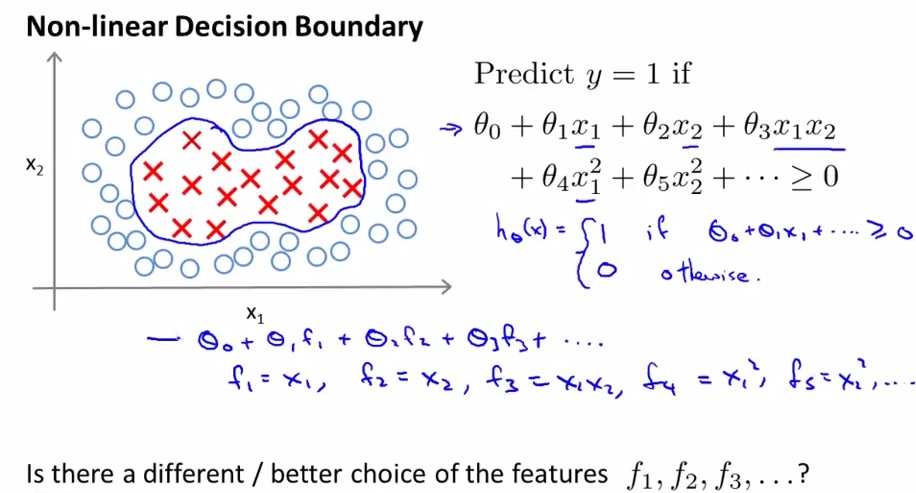• use fn to denotes the input polynomials
• Not clear if we even need high order polynomials. (Increase complexity, computationally expensive)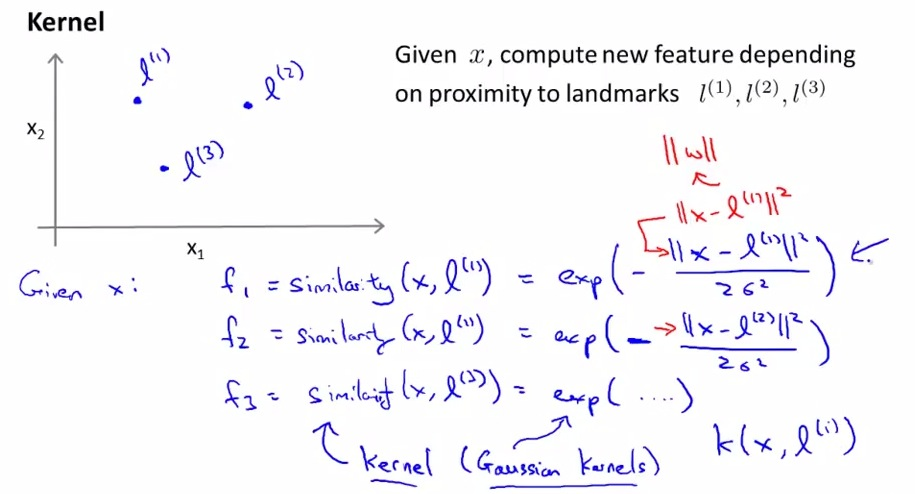• l = landmark. We choose let's say 3 landmarks.
• We calculate similarity by doing exponent of minus length of euclidean distance.
• Given examples of x. here's what we do. We are going to test how similar x to landmarks.
• More x approach the landmark, more similar x to landmark
• More similarity , more fi approach 1
• inside square = square of length Euclidean distance
• The kernel that we are using is actually more specific Gaussian kernels. and we simplify the similarity formula by calling k(x,l)
• What is it actually do??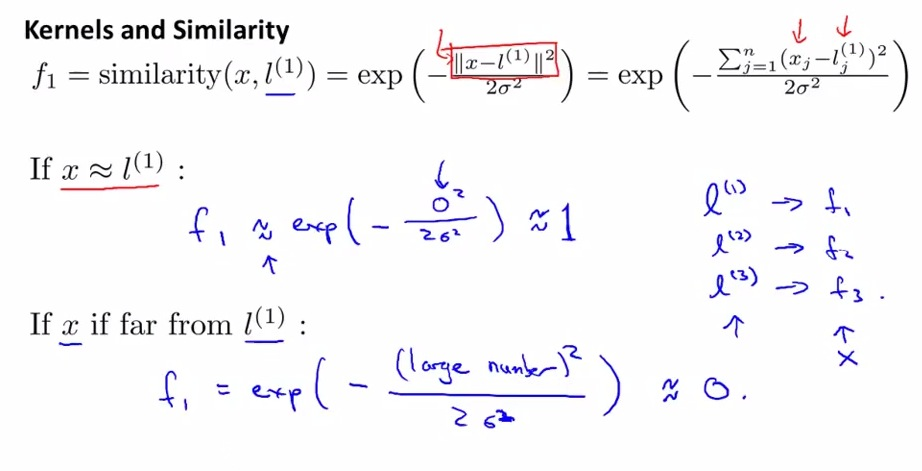• We are calculating the similarity of landmarks, on every x example.
• Then we calculate whether f1 approach 1 or approach zero.
• Given x, and 3 landmarks, we can produce 3 new set of features, based on x1 and x2.
• So that's it ini exponential function, next about the similarity function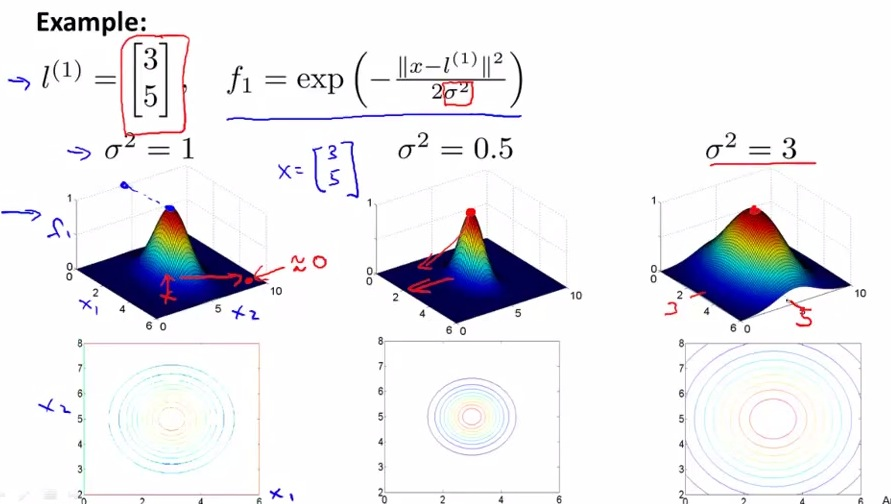• The vertical height is the value of f1. The height is at (3.5) the highest, where f1 is equal to 1.
• the horizontal coordinate perfomed by (x1,x2) features of one training example. (3.5) is where landmarks found its highest
• This is the sigma squared in Gaussian kernel. And by varying the sigma value, we will get different graph.
• if sigma squared too narrow, it will fall pretty quickly. if the sigma is too big, then the value of f1 will fall down slowly as the landmarks further away from (3.5)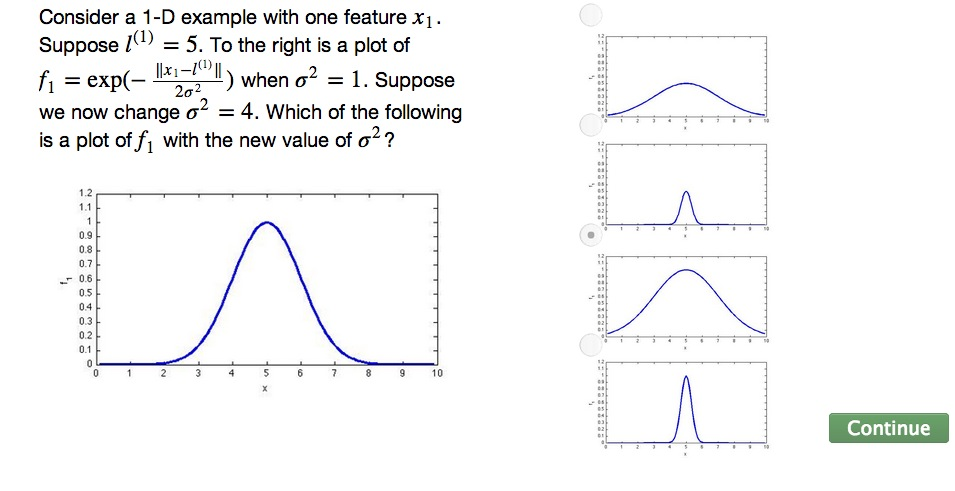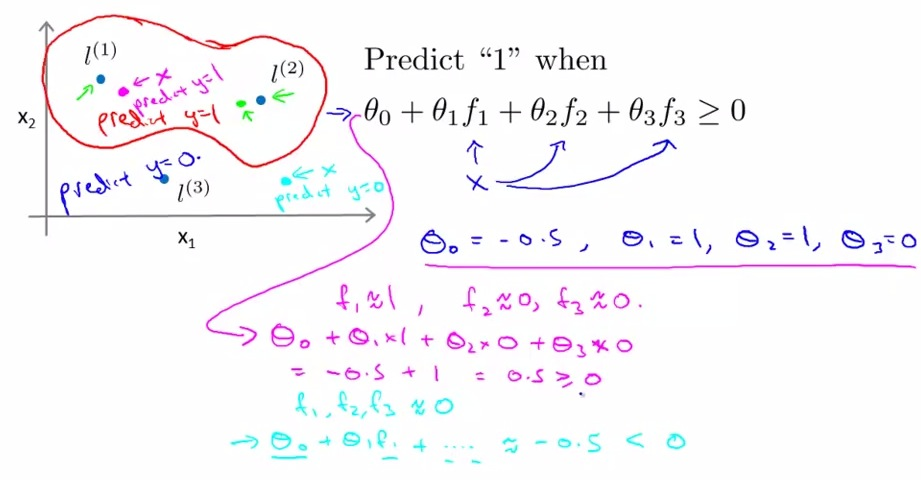• this is where we given training example x, and features of x1 and x2.
• The input value x in magenta is closer to f1 than the rest, so f1 = 1, rest is 0(f1 approach 1, rest approach 0). More similar, more approach to 1. With rest set zero, kernel end up computing theta0 and theta1 which equal 0.5, thus giving the prediction of y =1.
• The second input value x, not close to all three landmark, therefore f1,f2,f3 = 0. Then all that left is theta0 which will be resulting to -0.5, less than zero, and end up predicting y = 0.
• It will end up building a region that draw complex non-linear function that cover y =1 region, and rest is zero. By doing all that closer to l1 and l2, the region of y=1 is built.

• How do we choose the landmarks? Discussed next video
• Also putting together SVM and kernels?
• Are there also other similar function beside Gaussian kernels?
• Going to be solved how kernel can actually handle complex non-linear function